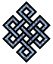fail2ban bad ip database: ip 220.120.106.254

| ip database | live view | stats | report | help | api key:

 ip: 220.120.106.254 hostname: 220.120.106.254 country:[KR] Korea, Republic of first reported: 05.10.2018 00:46.25 GMT+0200 last reported: 20.02.2019 05:29.28 GMT+0200 time period: 138d 05h 43m 03s total reports: 10 reported by: 4 host(s) filter(s): ssh (8) ssh (2) tor exit node no badips.com db Lookupport scan of '220.120.106.254':

[-hide]
```# Nmap 6.40 scan initiated Fri Oct  5 00:47:03 2018 as: /usr/bin/nmap -sU -sS -O 220.120.106.254
Nmap scan report for 220.120.106.254
Host is up (0.26s latency).
Not shown: 1000 open|filtered ports, 978 filtered ports
PORT      STATE  SERVICE
22/tcp    open   ssh
53/tcp    closed domain
80/tcp    open   http
443/tcp   closed https
554/tcp   closed rtsp
1935/tcp  closed rtmp
3690/tcp  closed svn
8443/tcp  open   https-alt
10003/tcp open   documentum_s
32772/tcp closed sometimes-rpc7
32778/tcp closed sometimes-rpc19
32782/tcp closed unknown
33354/tcp closed unknown
34572/tcp closed unknown
42510/tcp closed caerpc
44176/tcp closed unknown
49154/tcp closed unknown
49158/tcp closed unknown
49176/tcp closed unknown
50006/tcp closed unknown
50800/tcp closed unknown
56738/tcp closed unknown
No exact OS matches for host (If you know what OS is running on it, see http://nmap.org/submit/ ).
TCP/IP fingerprint:
OS:SCAN(V=6.40%E=4%D=10/5%OT=22%CT=53%CU=%PV=N%G=Y%TM=5BB6989B%P=x86_64-pc-
OS:linux-gnu)SEQ(SP=105%GCD=1%ISR=109%TI=RD%TS=U)OPS(O1=M564%O2=M564%O3=M56
OS:4%O4=M564%O5=M564%O6=M564)WIN(W1=2000%W2=2000%W3=2000%W4=2000%W5=2000%W6
OS:=2000)ECN(R=Y%DF=N%TG=FF%W=2000%O=M564%CC=N%Q=)T1(R=Y%DF=N%TG=FF%S=O%A=S
OS:+%F=AS%RD=0%Q=)T2(R=N)T3(R=N)T4(R=N)T5(R=Y%DF=Y%TG=80%W=0%S=O%A=S+%F=AR%
OS:O=%RD=0%Q=)T6(R=N)T7(R=N)U1(R=N)IE(R=N)

OS detection performed. Please report any incorrect results at http://nmap.org/submit/ .
# Nmap done at Fri Oct  5 00:47:55 2018 -- 1 IP address (1 host up) scanned in 53.65 seconds
```
```Σ = 68 | Δt = 0.0057001113891602s
```